# Co-separability of filter objects (Solved)

 Importance: Medium ✭✭
 Author(s): Porton, Victor
 Subject: Unsorted
 Keywords: filters
 Posted by: porton on: November 29th, 2009
 Solved by: Porton, Victor
Conjecture   Letandare filters on a set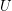and. Then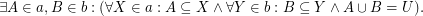See here for some equivalent reformulations of this problem.

This problem (in fact, a little more general version of a problem equivalent to this problem) was solved by the problem author. See here for the solution.

Maybe this problem should be moved to "second-tier" because its solution is simple.

## Bibliography

*Victor Porton. Open problem: co-separability of filter objects

* indicates original appearance(s) of problem.

### A counterexample?

From the link it seems you have proved the result. What about the following what seems to be a counterexample?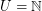(the set of integers),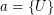,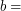the set of all infinite sets of integers

Now there is no set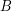that would be minimal in...

The set of all infinite sets of integers is not a filter. For example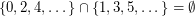.

### Correction

Sorry, I was too hasty. What I meant is thatis a "nontrivial ultrafilter" (wikipedia page ultrafilter) calls this "non-principal ultrafilter".

### No counterexamples, it is proved

Then take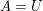and(I do not require filters to be proper).

Robert, why you are trying to find a counter-example for a proved theorem?

Here is the proof.

--
Victor Porton - http://www.mathematics21.org

### Is it really?

You require that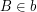, and my filterdoes not contain empty set. I'm trying to find a counter-example because either I misunderstand the statement of the theorem, or the theorem is false.

Some proofs just happen to have mistakes. Unfortunately, I don't understand yours, it apparently uses lot of notation (up, down, Cor, ...) that I'm unfamiliar with.

### Oh, my mistake

I made a mistake in the statement of the conjecture as published on OPG. I corrected the problem statement both on OPG and on my blog. It should berather than.

Indeed the equivalent reformulations of the theorem are correct and my proof (of a more general statement than this theorem) is not affected by the above mentioned error.

Robert, you do not understand me because I introduced new notations (that up, down, Cor, etc.) You may wish to read my preprint about these things (filters on posets and generalizations).